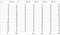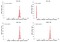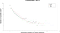# Hacking simple chaotic cipher

## Note: in this article I will talk about the work I did while studying at the University, so this paper is more educational than practical.

Since the late 90’s, a lot of dynamic chaos-based ciphers have been released, many of them have a fairly low strength. This paper analyzes one of these systems, published by Pareek in 2004.

Multiple one-dimensional chaotic maps is a cryptographic algorithm that implements block symmetric encryption.
Developed by Pareek, Patidar and Sud in 2004. It consists of four chaotic generators (logistic, tent, sine and cubic) that perform data protection in the order determined by the 128-bit key.

For encryption and decryption, the algorithm uses blocks of variable length, which are encoded by one of chaotic generatiors.

The algorithm can be divided into the initialization of the cipher and encryption/decryption of the data. Next, we will look at them briefly.

1. The secret key is divided into blocks K K … K.
2. Program calculates the initial conditions IC — starting seed for chaotic generators by the rule:
R = ΣK[i]/256, IC = R — [R], where [R] means rounding R with
dropping the fractional part(floor).
3. Algorithm initializes a linear recurrent sequence that controls chaotic maps switching:
Y[n+1] = (5*Y[n] + 1) mod 16
Y = [IC*100].

The encryption/decryption processis based on the pseudo-random sequence which is calculated as follows:

1. For all generators, X is assumed to be IC (X — initial state of the generator).
2. For a generator with index N=Y[n+1] mod 4 is performed IT=K[Y[n+1]] iterations, where K[Y[n+1]] is the key byte with index Y[n+1] (the value of Y[n+1] is determined by the control generator from Cipher Initialization section).
3. X[IT] is taken as the new X for this generator.
4. The next character in the sequence is Z = (X*1e5) mod 256.
5. The process generates B = Y[n+1] characters of control sequence(according to the block size), then chaotic generators are switched, and the process is repeated.

Next, the control sequence is added to the plaintext/ciphertext and we get
C[i] = (P[i]+Z) mod 256 for encryption
or
C[i] = (P[i]-Z) mod 256 for decryption.

The state of the control generator is calculated using the formula Y[n+1] = (5*Y[n] + 1) mod 16, this is a common recurrent sequence that will repeat after 16 steps.

The table below shows how the control generator Y[n] defines: the session key number is selected, the index of the chaotic generator used, and the number of bytes of plaintext that will be encrypted with it.The dependence of the session key number — B, the index of the chaotic generator — N, the number of blocks encrypted by each of the chaotic generators on Y.

The most important feature of the control generator for our cryptographic attack is a sufficiently large number of characters generated before changing its state vector. This feature allows us to examine blocks of 120 bytes in size and extract information about the current session key, block size, and index of the chaotic generator with an accuracy of 16 variants, which was used in this work.

Based on the analysis of the control generator was proposed the Multiple one-dimensional chaotic maps model which reflects the most important properties of the algorithm (the image below).

Encryption is performed over blocks of 120 bytes (see Analysis of the control generator section). The secret key is a set of session keys and the initial vector of the generator.

Main stages of the cryptographic algorithm:

1. The input receives a secret key (K, IC) and a plaintext block P..P.
2. Chaotic generators are initialized using IC.
3. The control generator based on IC generates a sequence of triples (i, B, N) that specify: the session key number, the block size, and the index of the chaotic map, respectively.
4. The map is selected from the block of chaotic generators. For each character of the control sequence, from a block of size B, K[i] chaotic map iterations are performed, then the result is truncated using the formula: Z=(X*1e5) mod 256. After that, the control sequence is fed to the masking block.
5. In the masking block, the control sequence and plaintext are added modulo 256, resulting in a ciphertext.

The dimension of the keys space Multiple one-dimensional chaotic maps is 2¹²⁸, which makes it practically impossible a brute force attack.
However, when studying the cipher model, attention was drawn to a rather small number of possible initial states of generators, which is only

where |K[i]| is a number of characters in the alphabet of the i-th byte of the key.

I also assumed that some of the control sequence characters are repeated, so that not all session keys are valid. To test the hypothesis, I wrote a python script. The script and example output are shown below.

During the experiment, it was found that the number of different characters of the control sequence generated at a certain fixed initial state of the generator and all possible session keys <256, which confirmed the hypothesis.

Statistics were obtained for the dependence of the number of initial states that generate a certain number of different characters in the control sequence (shown in the figure). The fewer different characters the generator generates, the more information can be obtained about the plaintext from the ciphertext, and the worse it protects the data.The number of initial vectors that generate a certain number of unique characters in the control sequence

In this paper, I propose a method for attack a Multiple one-dimensional chaotic maps based on known plaintexts, which implies that the cryptanalyst has a set of plaintexts and corresponding ciphertexts(to attack the cipher, you don’t need to know the whole documents, you just need to know some individual parts, for example, file headers or network transfer protocols have a predictable content). The task is to find a common encryption key in order to decrypt other messages with a similar key.

To obtain a secret key using plaintext and its corresponding ciphertext the following cryptanalysis scheme is proposed.

1. The control sequence is determined by subtracting the plaintext from the ciphertext using the formula: Z[i] = (C[i]-P[i]) mod 256.

2. All possible initial conditions are calculated using the formula:

Each IC[i] sets one of the 16 possible values of the control generator, so they can also be divided into subsets whose elements correspond to certain Y. Then each such subset is taken as current_states, and checked separately.

3. The control generator based on Y generates a sequence of pairs (i, N), where i is the sequence number of the current character of the control sequence, which varies from 0 to 119, and N — the index of the chaotic generator. N was used to calculate the current character of the control sequence.

4. The i-th set of states — current_states[i] is selected from the state catalog.

5. The i-th character — Z[i] is received from the gamma(control sequence) separation block.

6. A chaotic generator with index N (from a block of chaotic generators) iterates all values of X[j] from current_states[i], on all possible session keys, with deleting values that do not correspond to Z[i]. The result is a set of new states:

In the formula:

0 <=IT<256, IT ∈ ℤ and fIT iterations of the chaotic map with the index N and initial state X[j].

7. In the states filtering block, a set of duplicate states is deleted, i.e. those that meet the condition:

8. When the number of current states becomes small enough, we can start finding session keys: for each K[i] we find

with minimal number of keys, then we determine the set of their possible values K[i]

where f(N) is a chaotic map on step m,

X[m-1, k]∈ current_states[m-1] and X[m, l]∈ current_states[m].

9. K[i] uniquely identify the secret key — K=K..K, so we can reduce the set of keys for verification from 2 ¹²⁸ to ∏|K[i]|, i=1..16 variants, where |K[i]| is the number of possible values of the i-th session key. If this is not enough for attack, then we can go to the next block, using the current states of chaotic generators and current_states to determine Y. Also we can analyze the block again, iterating at step 6 using K[i](possible), first deleting the states which turned into invalid in the subsequent steps of attack.

The proposed cryptanalysis scheme was tested for a control sequence calculated on a random key. Tests have shown the effectiveness of the proposed attack: after studying the first block of data, the current states of chaotic generators are determined unambiguously, which allows us to fully determine the secret key when studying the next encrypted block.The process of filtering out values. The number of states remaining after processing the next character of the control sequence

So, this method for analyzing the Multiple one-dimensional chaotic maps allows us to uniquely determine the secret key by processing no more than 240 characters.

1. Simon Singh. The Code Book: The Science of Secrecy from Ancient Egypt to Quantum Cryptography. August 29, 2000.
2. Robert Churchhouse. Julius Caesar, the Enigma, and the Internet.
3. N. Pareek, V. Patidar, K. Sud. Cryptography using multiple one-dimensional chaotic maps . Elsevier 2005.
4. G. Álvarez, F. Montoya, M. Romera, G. Pastor. Cryptanalysis of a discrete chaotic cryptosystem using external key. Elsevier 2003.
5. D. Arroyo, G. Alvarez, S. Li. Some Hints for the Design of Digital Chaos-Based Cryptosystems: Lessons Learned from Cryptanalysis.
6. Jun Wei , X. Liao, K. Wong, T. Zhou. Cryptanalysis of a cryptosystem using multiple one-dimensional chaotic maps. Elsevier 2007.
7. S. Li , G. Alvarez, Z. Li, W. Halang. Analog Chaos-based Secure Communications and Cryptanalysis: A Brief Survey.
8. W. Kahan . Lecture Notes on the Status of IEEE Standard 754 for Binary Floating-Point Arithmetic. 1997.